Courses
Courses for Kids
Free study material
Free LIVE classes
More

# How to Write 10?LIVE
Join Vedantu’s FREE Mastercalss

## Overview of Number 10

Are you still surfing the web to find out more about how to make your child learn to memorize the number 10? If so, you have now reached the appropriate spot because, in this article, the information about the number 10 is discussed deeply by including the number 10 activities, and number 10 preschool worksheets. The writing makes use of several attractive images to help the students to retain the topic for a longer time. So, now let us begin with the topic.

## What is the Number 10?

The natural number 10 is the one that comes after 9 and before 11, making it a number, numeral, and digit. It has greater significance in mathematics, especially decimals. It is the first even two-digit number, which is also a composite number. The divisors and factors of 10 are 1, 2, 5, 10.

## Number 10 With Objects

The counting number discussed in the article is ten. The objects, namely leaves, are used to depict the number 10 in the image provided below. Parents and teachers get benefits in teaching their children the number ten with the help of these illustrations.Number 10 with Objects

## How to Write 10?

One should continuously practice writing the number 10, on the dotted line by using the tracing technique. Doing so will help the children learn the method of writing the number 10. The worksheet given below is based on the idea of the dotted and tracing concept that will certainly help kids learn how to write 10.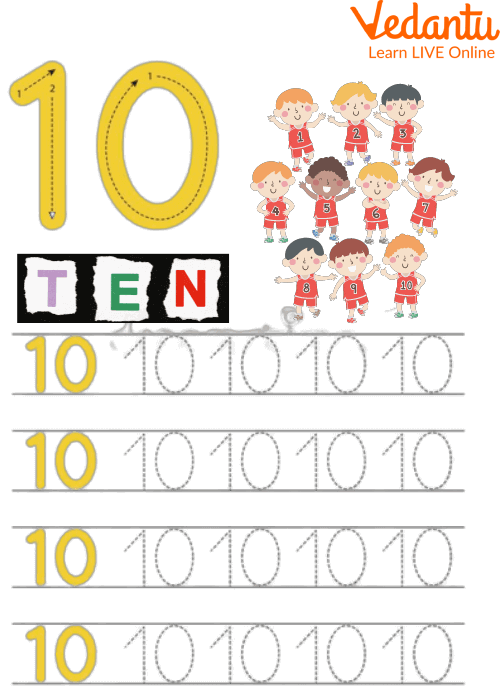How to Write 10?

## Writing Number 10 in Different Formats

The number 10 can be written in different writing forms, such as:

• In number names

The number 10 is represented as ten when written in number names.

• In ordinal form

In terms of ordinal form, the number 10 is written as tenth or 10th.

In roman numeral form, the number 10 is depicted by writing the symbol X.

In terms of the square root of a number, the number 10 is written as the square root of the number 100, i.e.$\sqrt{100}$.

## Solved Examples

Q 1. Represent the number 10 on the number line.

Ans. Follow the given steps to represent the number 10 on the number line:

• Draw a straight line of any length using the scale.

• Represent the integers on the number line.

• Mark the point where you get the number 10.

Thus, the number 10 is represented on the number line.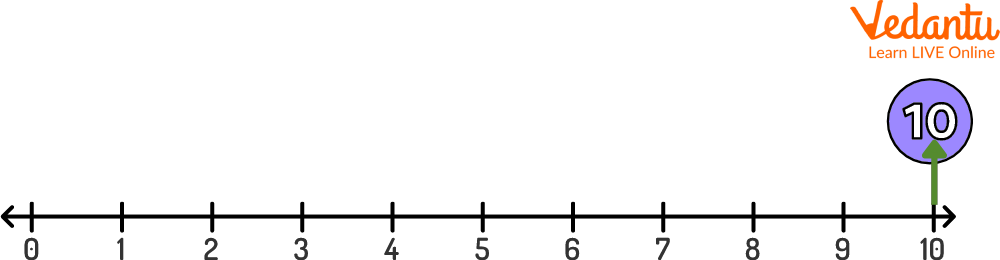Number 10 on the Number Line

Q 2. Write the numbers from 1 to 10.

Ans. 1, 2, 3, 4, 5, 6, 7, 8, 9, and 10 represent the writing numbers 1 to 10. These numbers are generated by adding 1 to the preceding terms.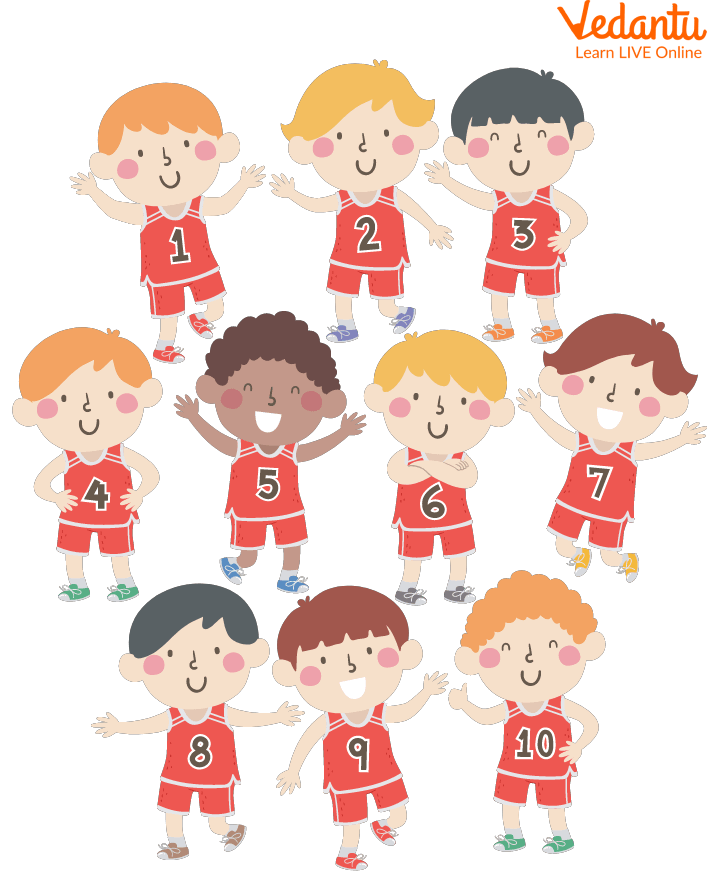Writing Numbers to 10

## Practice Problems

By this time, children would have gained a clear picture of the number 10 and the other numbers in their minds. To evaluate their progress, let us assign them some practice problems based on number 10 activities for preschool.

Q 1. Circle the correct number of objects shown on the left side of the image.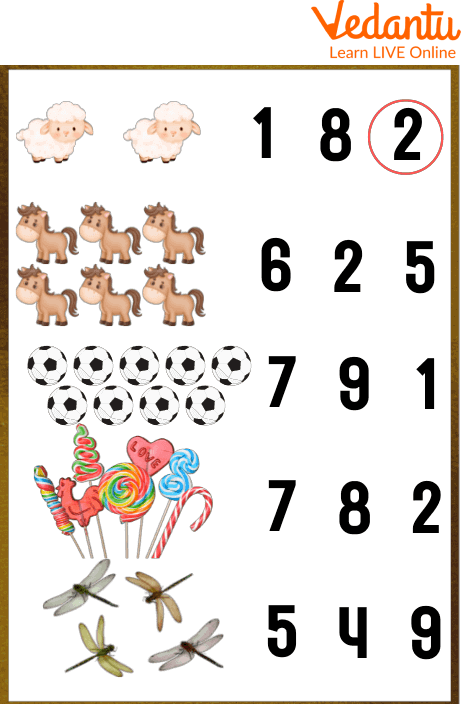Number 10 Activities, Count and Write

Q 2. Circle the number 10 in the below image.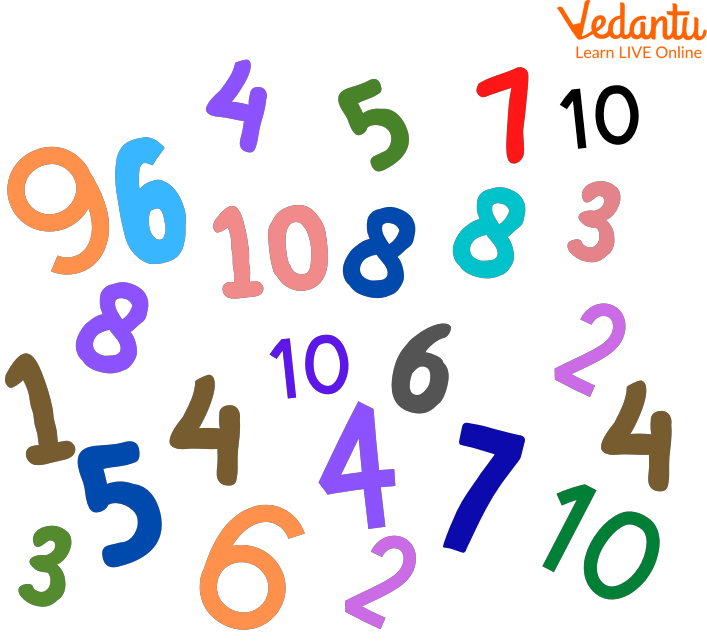Number 10 Activities, Circle the Number 10

## Number 10 Preschool Worksheets

Some of the Number 10 Preschool Worksheets are given below that need to be completed by the kids alone without any help from others.

Q 1. Write the missing digit number in the box by completing the counting from 1 to 10.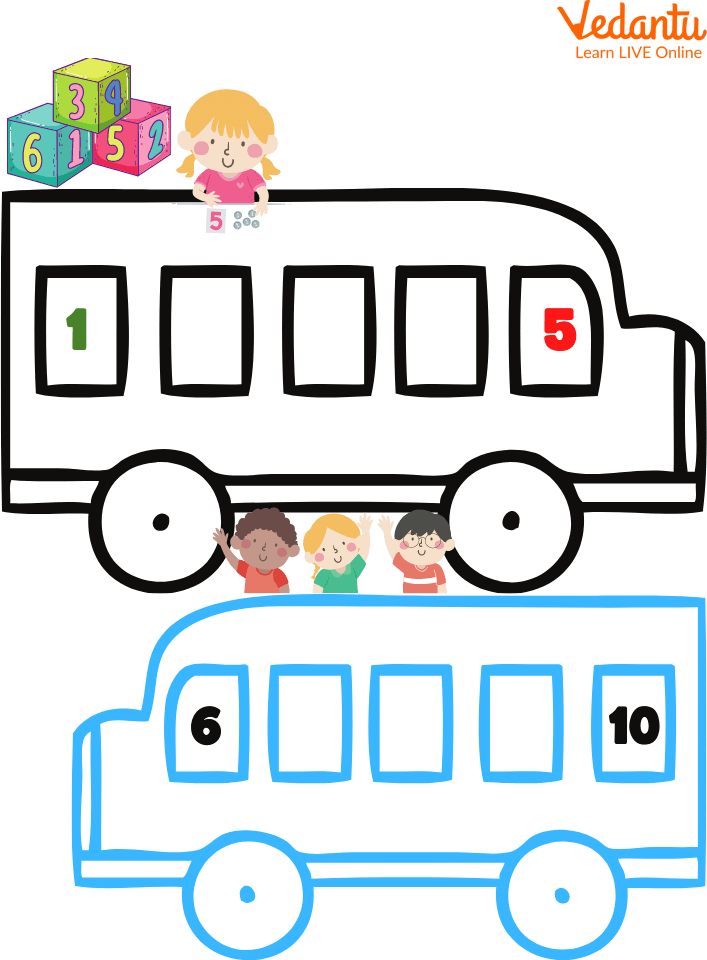Writing Numbers to 10

## Summary

To summarize here, the concept of the number 10. Counting numbers is the fundamental building block of mathematics. This article has covered every topic related to the number 10, along with the number 10 activities for preschool, by using numerous attractive images and simple language, for a better understanding of the concepts by the children. Wishing you find the article beneficial in learning the number 10 and you enjoy reading it. Always feel comfortable asking your doubts, if any, in the comment section given below.

Last updated date: 30th Sep 2023
Total views: 95.7k
Views today: 2.95k

## FAQs on How to Write 10?

1. In geometry, what do we call a polygon having ten sides?

In geometry, we use the term decagon to represent a polygon having ten sides. This is because 10 is sometimes also called a decagonal number.

2. What is the main use of 10 in the decimal number system?

The number 10 has a wide application in the decimal number system. The prime of all the applications is that it is used as the base number in the decimal number system.

3. Can one express 10 as the sum of the first three prime numbers?

Yes, one can always express 10 as the sum of the first three prime numbers. The first three prime numbers are given by 2, 3, and 5; whose sum is equal to the number 10. So, the number 10 is equal to the sum of these prime numbers.